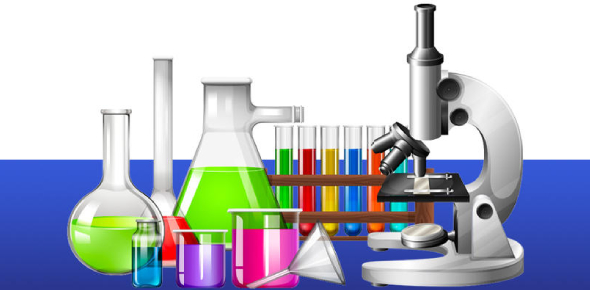# Chemistry Trivia Test: Ultimate Quiz!

10 Questions | Total Attempts: 47SettingsDo you have any chemistry knowledge? Chemistry is a science, which means that experiments are conducted, and the scientific method is followed. Chemistry is the study of matter, its properties, and interactions with other matter. It is a natural law that examines the changes made in materials or substances. In chemistry, you earn the relationship between chemistry and energy. Don’t let your brain explode. You can pass this chemistry trivia quiz. Go for it.

• 1.
Which of the following has high electronegative?
• A.

Oxygen

• B.

Carbon

• C.

Hydrogen

• D.

Florine

• E.

Chlorine

• 2.
Hydrogen bonding is developed due to:
• A.

Low temperature

• B.

Low temperature

• C.

Ionic character of covalent bonding

• D.

Sharing of electron

• E.

Low electronegativity

• 3.
Neutron was discovered by:
• A.

J.J Thomson

• B.

• C.

Rutherford

• D.

Bohr

• E.

None of these

• 4.
Which of the following has sp3 hybridization?
• A.

CH4

• B.

C6H6

• C.

C2H2

• D.

C4H8

• E.

None of these

• 5.
How many electrons are there in H+ (hydrogen ion)?
• 6.
In Methane C-H bond angle is?
• A.

190.5

• B.

120

• C.

150

• D.

90

• E.

180

• 7.
NH4(Ammonium) is an example of:
• A.

Covalent bond

• B.

Co-ordinate covalent bond

• C.

Hydrogen bond

• D.

Ionic bond

• E.

None of these

• 8.
At S.T.P H2O(water) is liquid due to:
• A.

Hydrogen bond

• B.

Bond distance between H & C

• C.

Covalent bond

• D.

Bond energy

• E.

None of these

• 9.
At S.T.P, the compounds having Covalent bonding are mostly:
• A.

Gases

• B.

Liquid

• C.

Solid

• D.

Wax like shape

• E.

None of these

• 10.
Hydrogen is placed at:
• A.

1A

• B.

2A

• C.

3B

• D.

7A

• E.

Its position is decided

Related TopicsBack to top